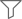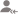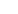Find the best tutors and institutes for Class 10 Tuition

Find Best Class 10 Tuition

Please select a Category.

Please select a Locality.

No matching category found.

No matching Locality found.

Outside India?

Search for topics

# Class 10 Tuition Updates

Ask a QuestionAll

Lessons

DiscussionLesson Posted on 28/09/2018 CBSE/Class 9/Mathematics/Unit I: Number systemsDeepa Thakur Sharma

I have always loved teaching and would give personal attention to each student and do not mind repeating...

NUMBER A mathematical symbol represented by a set of digits is called a number.we can express a number in two, i.e., in words & in figures.e.g., if a number is 3290, then we can write this number in words as 'three thousand two hundred and ninety.' DECIMAL SYSTEM In our daily life, we use numbers... read more

NUMBER

A mathematical symbol represented by a set of digits is called a number.we can express a number in two, i.e., in words & in figures.e.g., if a number is 3290, then we can write this number in words as 'three thousand two hundred and ninety.'

DECIMAL SYSTEM

In our daily life, we use numbers everywhere for counting, for measuring weight, for measuring height, for scores of games etc. In the decimal system, we use ten symbols 0,1,2,3,4 5,6,7,8 and 9 which are called digits. Here, 0 is called the insignificant number, and the rest digits are called significant digit.

read less
Comments 1
Dislike Bookmark Share

Lesson Posted on 24/09/2018 CBSE/Class 9/Mathematics/Unit I: Number systems/Real NumbersKanika

Taught class 9-10 maths and science in coaching centre and giving home tuition to classes 6-8 for all...

--------------To find four rational numbers between 3 and 5 Solution: As we have to find four rational numbers between 2 nos then add 4+1 in the denominator 3*5/1*5= 15/5 5*5/1*5=25/5 So we can write any four numbers between 15/5 and 25/5 16/5, 17/5, 18/5, 19/5 Problem2) To find five rational... read more

--------------
To find four rational numbers between 3 and 5

Solution: As we have to find four rational numbers between 2 nos then add 4+1 in the denominator

3*5/1*5= 15/5

5*5/1*5=25/5

So we can write any four numbers between 15/5 and 25/5

16/5, 17/5, 18/5, 19/5

Problem2) To find five rational numbers between 5/7 and 6/7

Solution: As both have the same denominator so we can divide and multiply both the numbers by 10

5*10/7*10= 50/70

6*10/7*10=60/70

Therefore five rational numbers are 51/70, 52/70, 53/70, 54/70, 55/70

read less
Comments
Dislike Bookmark Share

Lesson Posted on 24/09/2018 CBSE/Class 10/Mathematics CBSE/Class 9/Mathematics/Unit I: Number systems/Real NumbersKanika

Taught class 9-10 maths and science in coaching centre and giving home tuition to classes 6-8 for all...

there are different types of numbers 1) Whole Number: the numbers which start from 0 eg, 0,1,2,3,..... Upto infinity . They are denoted by W. They are positive number 2) Natural Number: The numbers which start from 1 i.e. 1,2,3...... Upto infinity. They are denoted by N. They also include only positive... read more

there are different types of numbers

1) Whole Number: the numbers which start from 0 eg,  0,1,2,3,..... Upto infinity . They are denoted by W. They are positive number

2) Natural Number: The numbers which start from 1 i.e. 1,2,3...... Upto infinity. They are denoted by N. They also include only  positive number

3) Integers: It contains positive and negative numbers both,  also include zero. Eg,  -1,-26,0,5,45,etc. They are denoted by Z

4) Rational Number: Number which can be written in p/q form where p and q both are integers and q should not be equal to zero.

Eg,   -3 yes it is a rational  number because it can be written in p/q form as -3/1

They are denoted by Q

read less
Comments
Dislike Bookmark Share

Overview

Questions 78

Lessons 21

Total Shares12,434 Followers

Lesson Posted on 24/09/2018 CBSE/Class 9/Mathematics/Unit I: Number systems CBSE/Class 9/MathematicsKanika

Taught class 9-10 maths and science in coaching centre and giving home tuition to classes 6-8 for all...

Whole number can not be a Natural Number because whole number starts from 0 while Natural number starts from 1 which des not includes zero as it not include zero so it can not be a whole number. Natural number can be a whole number because Natural number starts from 1 and whole number starts from 0... read more

Whole number can not be a Natural Number because whole number starts from 0 while Natural number starts from 1 which des not includes zero as it not include zero so it can not be a whole number.

Natural number can be a whole number because Natural number starts from 1 and whole number starts from 0 which includes 1

Natural no: 1,2,3,4,5,.....

Whole no: 0,1,2,3,4,5,......

The bold numbers which are presented in the whole number are nothing but the natural numbers except 0

Therefore these 1,2,3,4,5,.... are the natural numbers which are coming in the whole numbers

Integer can be a Rational number because every integer can Be expresses as p/q form eg: 3 is integer can be expressed as 3/1

-4 is an integer and can be written as -4/1 where p is -4 and q is 1

Rational number can not be an Integer because 2/5 is not an integer but it is a rational number as p is 2 and q is 5 but we know that integer does not have p/q form.

read less
Comments
Dislike Bookmark Share

Lesson Posted on 17/09/2018 CBSE/Class 9/Mathematics/Unit 2-Algebra/Linear equations in 2 variablesTapas Dey

I am an experienced, qualified tutor with 1 year of experience in teaching Maths and Science, in State...

Check Which of the following are solutions of the equation x-2y=4 and which are not. i. (0,2) ii. (2,0) iii. (4,0) iv. (1,1) Ans: i). (0,2) Putting x=0, and y= 2 in the L.H.S of the give equation, x-2y=0-2*2=-4 ≠4 L.H.S≠R.H.S Therefore, (0,2) is not a solution of this equation. ii. (2,0) Putting... read more

Check Which of the following are solutions of the equation x-2y=4 and which are not.

i. (0,2) ii. (2,0) iii. (4,0) iv. (1,1)

Ans:

i). (0,2)

Putting x=0, and y= 2 in the L.H.S of the give equation,

x-2y=0-2*2=-4 ≠4

L.H.S≠R.H.S

Therefore, (0,2) is not a solution of this equation.

ii. (2,0)

Putting x=2, and y= 0 in the L.H.S of the give equation,

x-2y=2-2*0=2 ≠4

L.H.S≠R.H.S

Therefore, (2,0) is not a solution of this equation.

iii. (4,0)

Putting x=4, and y= 0 in the L.H.S of the give equation,

x-2y=4-2*0=4 =4

L.H.S=R.H.S

Therefore, (4,0) is a solution of this equation.

iv. (1,1)

Putting x=1, and y= 1 in the L.H.S of the give equation,

x-2y=1-2*1=-1≠4

L.H.S≠R.H.S

Therefore, (1,1) is not a solution of this equation.

read less
Comments
Dislike Bookmark Share

Answered on 21/10/2018 CBSE/Class 10 CBSE/Class 9/MathematicsChandan Kumar

Tutor

3000 per month
Answers 6 Comments
Dislike Bookmark Share

Answered on 13/08/2018 CBSE/Class 10 CBSE/Class 9/MathematicsKalaiRaj

350 / hour 5000 -6000 per month
Answers 5 Comments
Dislike Bookmark Share

Answered on 05/08/2018 CBSE/Class 9/Mathematics CBSE/Class 10/Mathematics/UNIT II: Algebra/PolynomialsCamellia Ray

Tutor

A very good thought Moumita. See different boards and different school have different criteria, plan accordingly. 1st of all keep few things in mind *To motivate and encourage the students, many students have fear in the subject so teach in such a way so that they are able to overcome their fears.... read more

A very good thought Moumita. See different boards and different school have different criteria, plan accordingly.

1st of all keep few things in mind

*To motivate and encourage the students, many students have fear in the subject so teach in such a way so that they are able to overcome their fears.

*Never scold them because if they ate scared they will never ask the doubts.

*Follow reference books like for cbse R.D.SHARMA&R.S.AGGARWAL etc.

* Motivate them to give olympiad exam.

*Make different set of question papers and take test once the chapter is finished.

*Discuss their monthly performance with parents.

All the best.

read less
Answers 3 Comments 1
Dislike Bookmark Share

Answered on 04/08/2018 CBSE/Class 9/Mathematics CBSE/Class 11

I want to teach to class 9&10Ravi Ranjan S.

Tutor

Please takeany membership
Answers 2 Comments
Dislike Bookmark Share

## Class 10 Tuition in:

Answered on 11/01/2018 CBSE/Class 9/Mathematics Tuition/Class IX-X TuitionD Mukherjee

Teacher

In total there are 5 children out of which 3 are girls So the probability of selecting a girl is 3/5 or 0.6 or 60 %
Answers 9 Comments
Dislike Bookmark Share

## About UrbanPro

UrbanPro.com helps you to connect with the best Class 10 Tuition in India. Post Your Requirement today and get connected.

x

Ask a Question

Please enter your Question

Please select a Tag

UrbanPro.com is India's largest network of most trusted tutors and institutes. Over 25 lakh students rely on UrbanPro.com, to fulfill their learning requirements across 1,000+ categories. Using UrbanPro.com, parents, and students can compare multiple Tutors and Institutes and choose the one that best suits their requirements. More than 6.5 lakh verified Tutors and Institutes are helping millions of students every day and growing their tutoring business on UrbanPro.com. Whether you are looking for a tutor to learn mathematics, a German language trainer to brush up your German language skills or an institute to upgrade your IT skills, we have got the best selection of Tutors and Training Institutes for you. Read more